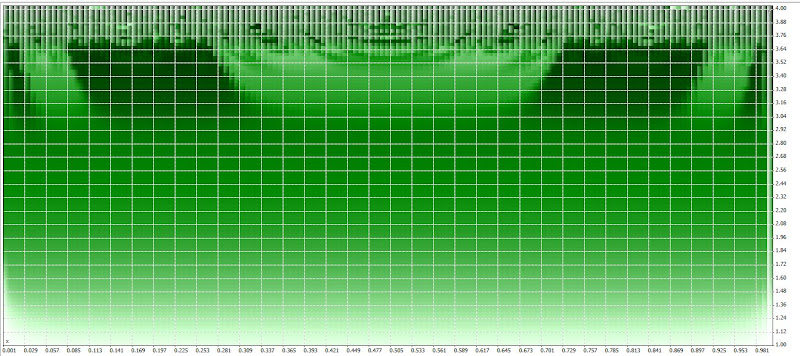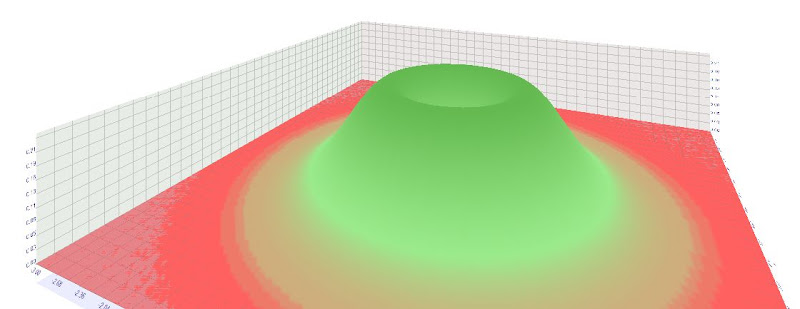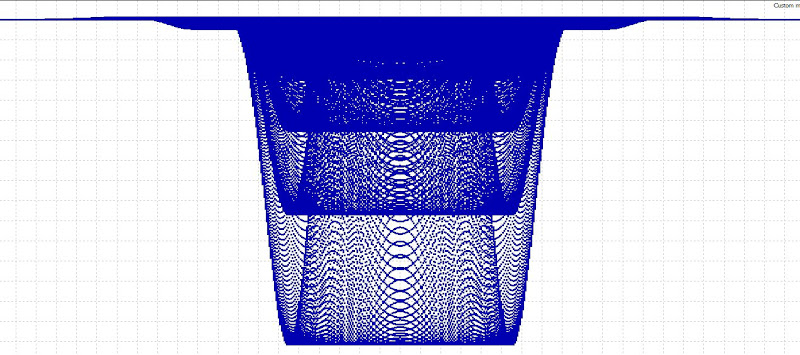• ### Chaos kernel function using the logistic map?

Revision created 3333 days ago by JohnLast

• ### Chaos kernel function using the logistic map?

Revision created 3333 days ago by JohnLast

• ### Chaos kernel function using the logistic map?

Revision created 3333 days ago by JohnLast

• ### Chaos kernel function using the logistic map?

Revision created 3351 days ago by JohnLast

• ### Chaos kernel function using the logistic map?

Revision created 3351 days ago by JohnLast

• ### Chaos kernel function using the logistic map?

Revision created 3351 days ago by JohnLast

• ### Chaos kernel function using the logistic map?

Revision created 3351 days ago by JohnLast

• ### Chaos kernel function using the logistic map?

Revision created 3351 days ago by JohnLast

• ### Chaos kernel function using the logistic map?

Revision created 3351 days ago by JohnLast

• ### Chaos kernel function using the logistic map?

Revision created 3351 days ago by JohnLast

## Chaos kernel function using the logistic map?: Revision

### Chaos kernel function using the logistic map?

Last updated 3333 days ago by JohnLast

I made some experiments with different kernels and I would like to share among friends some ideas.

Well it is about to use a function expressing chaotic behaviour itself as a kernel.

The simplest thing was to look at the most simple logistic map.That is the equation of the logistic map. What we can do with that.

What is interesting for us is the parameter r. If we vary the parameter we will have different behaviour (sensitivity of the initial conditions). I keep it short here but we can get some very interesting  stretching-and-folding structures , please look at the Wikipedia article.От Поле за пускане

#property version "1.00"
//--- input parameters
input double x=0.5;

input double r=1;
//+------------------------------------------------------------------+
//| Tester function |
//+------------------------------------------------------------------+
double OnTester()
{
//---

double w0 = r*x*(1-x);
double w1 = r*w0*(1-w0);
double w2 = r*w1*(1-w1);
double w3 = r*w2*(1-w2);
double w4 = r*w3*(1-w3);
//---
return(w2);
}

This is the code that can be used to make mathematical simulations in Metatrader 5.

Here I do not use for and while operators, because I want the code to be mathematically explicit for the non coders here.

Well what we can do next and yes we can replace the x by the gaussian kernel (the gaussian kernet iteslf has x and y and the output varies from 0 to 1). So it is fine let take a look what we get.От Поле за пускане

The code is here:

#property version "1.00"
//--- input parameters
input double x=-3.0;
input double y=-3.0;
input double r=1;
//+------------------------------------------------------------------+
//| Tester function |
//+------------------------------------------------------------------+
double OnTester()
{
//---

double w0 = MathExp(-x*x-y*y);
double w1 = r*w0*(1-w0);
double w2 = r*w1*(1-w1);
double w3 = r*w2*(1-w2);
double w4 = r*w3*(1-w3);
//---
return(w2);

So in the chaos kernel we have 3 parameters (look at the comments). x and y are coming from the gaussian kernel the r is from the logistic map equation.

But there is something more, lol, look at the recurrence relation, we can use the first cycle w1, the second, w2, the thirs w3 (it is up to us to choose how far we can go). So w2 is more complex that w0 and w3 has a more complex structure than w2.

The logistic map stretching-and-folding structures combined with an input coming gaussian kernel are able to produce weird things lol.От Поле за пускане

So can we use this as a kernel? The r parameter is the kernel's chaotic parameter than can be limited or expanded.

In my imagination the real science fiction artificial intelligence need to use some kind of chaotic parameter, stepping from the deterministic to the non deterministic.

The question is:

Can we use kernels with chaotic behavior to model chaotic systems?

I think yes too, if that is true that is another kernel trick.